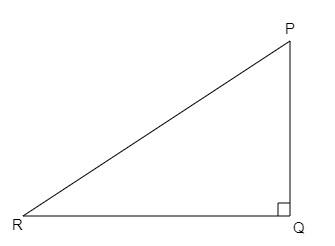# In $\Delta P Q R$, right-angled at $Q, P Q=3 \mathrm{~cm}$ and $P R=6 \mathrm{~cm}$. Determine $\angle P$ and $\angle R$.

Given:

In $\Delta P Q R$, right-angled at $Q, P Q=3 \mathrm{~cm}$ and $P R=6 \mathrm{~cm}$.

To do:

We have to determine $\angle P$ and $\angle R$.

Solution:In $\Delta P Q R$,

$sin\ R=\frac{PQ}{PR}$

$=\frac{3}{6}$

$=\frac{1}{2}$

$=\sin 30^{\circ}$          (Since $\sin 30^{\circ}=\frac{1}{2}$)

$\Rightarrow R=30^{\circ}$

We know that sum of the angles in a triangle is $180^o$.

Therefore,

$\angle P+\angle Q+\angle R=180^o$

$\angle P+90^o+30^o=180^o$

$\angle P=180^o-120^o$

$\angle P=60^o$

Hence, $\angle P=60^o$ and $\angle R=30^o$.

Updated on: 10-Oct-2022

40 Views